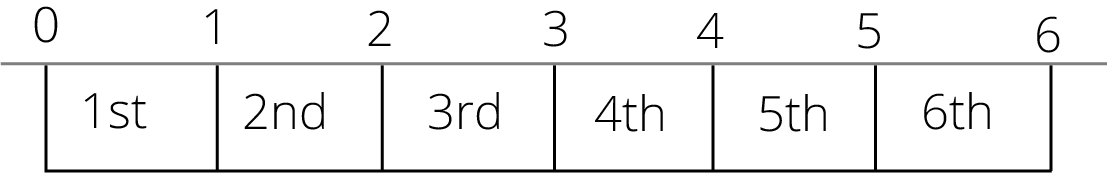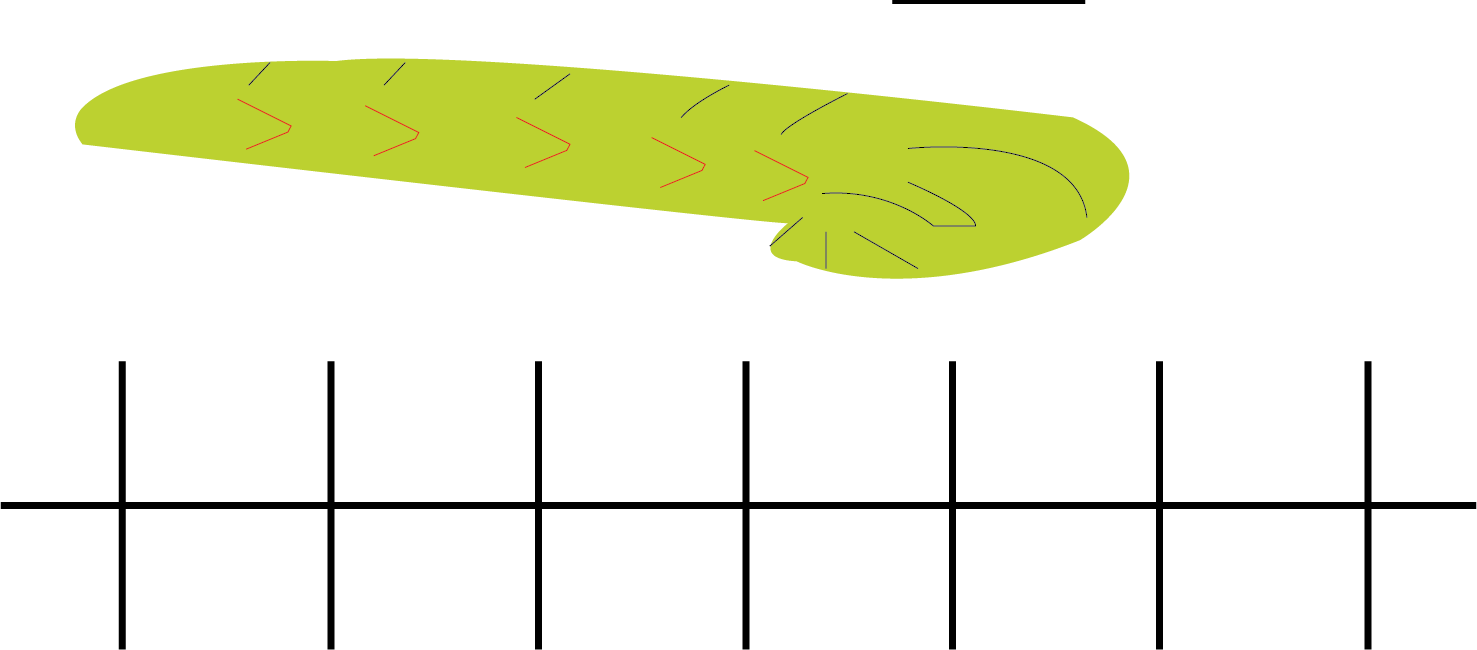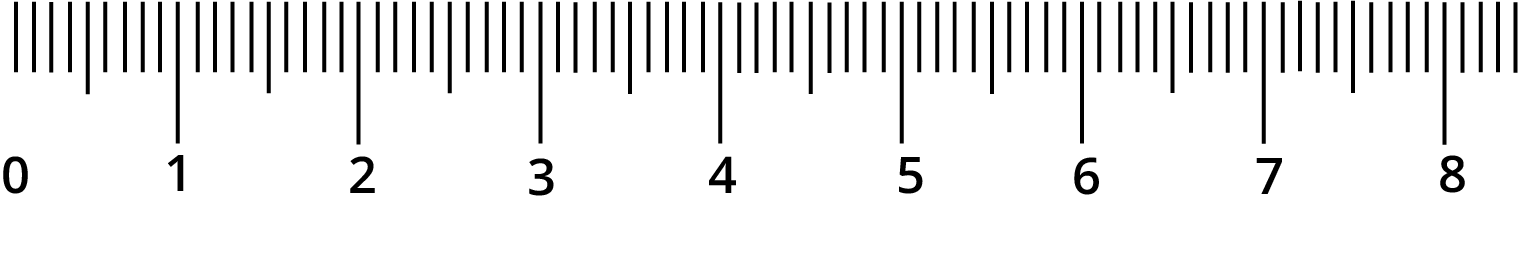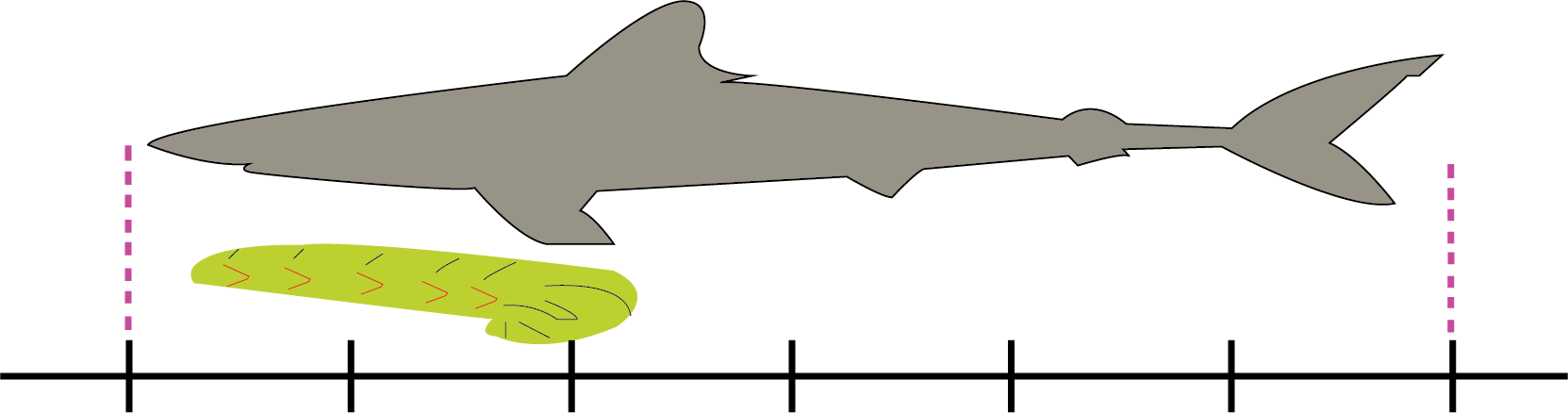Courses
Courses for Kids
Free study material
Free LIVE classes
More

# NCERT Revision Notes for Class 3 Maths Chapter 4 Long and ShortLIVE
Join Vedantu’s FREE Mastercalss

## NCERT Maths Class 3 Chapter 4 Long and Short Revision Notes

Your exam preparation will become a lot easier with the Long and Short revision notes formulated by the top subject experts at Vedantu. It is time to add these notes to your study material to get a concise understanding of the basic concepts of lines and lengths.

The revision notes for CBSE Class 3 Maths Chapter 4 Long and Short will teach you how to measure lines using a centimeter scale. It will also teach how to interconvert the length units and compare them. Download these revision notes in PDF format for free and refer to them at your convenience.

Also, check CBSE Class 3 Maths revision notes for other chapters:

Last updated date: 01st Oct 2023
Total views: 114.3k
Views today: 3.14k

## Revision Notes Class 3 Mathematics Chapter 4 - Long and Short

### Long and Short

• If you measure the length of the table with your hand it will count more.

• You can say measuring with your hand will take more units while measuring the table compared with your father’s hand.

## What is a Unit?Units Representation

• Here are 6 units, 1, 2, 3, 4, 5 and 6.

• If you jump from 0 to 1 or 1 to 2 or 2 to 3, it will be 1 unit.

• The object which used more units is long.

• The object which used fewer units is short.

Solved Example 1: How many units long is the caterpillar?Length of Caterpillar

Ans: By counting the length of the caterpillar is 4 units.Measurement Scale

• Every digit from 0 to 8 is at one centimetre.

• In one centimetre there are 10 small sections.

• Each small section is one millimetre.

• 10 millimetres is equal to 1 centimetre.

Solved Example 1: how many millimetres are there in 2 centimetres?

Ans: we know that there are 10 millimetres in 1 centimetre.

So, there will be 20 millimetres in 2 centimetres.

## Centimetre versus Millimetre

 Centimetres Millimetres 1 10 2 20 3 30 4 40 5 50

## What is a Centimetre?Length Comparison of the Shark and the Caterpillar

• Here the caterpillar is 4 centimetres long.

• The baby shark is 13 centimetres long.

Solved Example 1: Which one is long and which is short?

Ans: Baby sharks are long and caterpillars are short.

Solved Example 2: which is mapping more units in the above image?

Ans: As we know, a longer object takes more units so a baby shark is mapping more units.

• You never come to know exactly how long an object is if you measure it with rope, hand, shoe-string, thread etc.

• For an exact measurement, always use a measuring tape or a measurement rod.

## How Long is a Metre?

• You know, there are 100 centimetres in 1 metre.

• A measurement rod is 1 metre long and it is used by shopkeepers to measure clothes.

• Here are some objects listed which are longer than or shorter than 1 metre

 Longer than 1 metre Shorter than 1 metre Length of bed Length of books Width of bedroom Length of shoe Length of whiteboard used in the classroom Length of writing pen Length of see-saw in children’s park Height of puppies Length of school bus Length of the TV remote
• Now there are millimetres, centimetres and metres.

• 1 millimetre is shorter than 1 centimetre.

• 1 centimetre is shorter than 1 metre.

Solved Example 1: which unit is used to measure the length of a mobile phone?

Ans: centimetre

Solved Example 2: which unit is used to measure the length of a car?

Ans: metre

## Centimetres or Metres?

• For shorter objects, we will use centimetres as a unit.

• For longer objects, we will use metres as a unit.

• o measure the length of shoes we will use centimetres as a unit.

• To measure the distance between your home to the school we will use metres as a unit.

 Measured in Centimetre Measured in Metre Waist of your friend Height of the school gate Length of drum stick Length of yoga mat Height of cat Height of building Width of your hand Length of the cricket pitch Length of your fingers Length of the train

Solved Example 1: How wide can the thumb be, 1 millimetre or 1 centimetre or 1

metre?

Ans: The width of the thumb can be 1 centimetre.

Solved Example 2: What can be the length of the pen 10 millimetres or 10 centimetres or 10 metres?

Ans: The length of the pen can be 10 centimetres.

Solved Example 3: How long can the sari be, 5 millimetres or 5 centimetres or 5 metres?

Ans: The length of the sari can be 5 metres.

## Metres or Kilometres?

• For shorter distances, we will use metres. For example, the length and width of classrooms, halls, bedrooms, etc.

• For longer distances, we will use kilometres. For example, the distance between two cities. The distances between your home to your school.

• There are 1000 metres in 1 kilometre.

Solved Example 1: The distance between two railway stations can be, 10 metres or 10 kilometres.

Ans: The distance between two railway stations can be 10 kilometres.

Solved Example 2: which one is longer, the distance between your home and your friend's home is 200 metres or the distance between two cities is 200 kilometres?

Ans: The distance between two cities is longer than the distance between your home and your friend’s home.

## Practice Problem

Question 1. Which animal has the longest and which has the shortest tail among monkey, rat, pig and house lizard?

Question 2. Match the following

 Object Length or Distance 1. Width of the writing pad a) 100 kilometres 2. Distance between two cities b) 30 centimetres 3. Width of the classroom c) 18 centimetres 4. Width of bed d) 6 metres 5. Length of your hand e) 2 metres

Ans:

1. Monkey has the longest tail and the house lizard has the shortest tail.

 Object Length or Distance 1. Width of the writing pad b) 30 centimetres 0. Distance between two cities a) 100 kilometres 0. Width of the classroom d) 6 metres 0. Width of bed e) 2 metres 0. Length of your hand c) 18 centimetres

## Importance of NCERT Class 3 Maths Chapter 4 Revision Notes

The subject matter experts have curated and prepared these revision notes following the CBSE guidelines. These revision notes cover the entire chapter in a precise way. The importance of these revision notes for Class 3 students is as follows.

• It comprises an easy explanation of the fundamental concepts of length and measurement of lines.

• Students will find out how to compare lines and comprehend the sums of this chapter from these revision notes.

• They will also comprehend the terms used in the sums given in the exercises and learn how to solve them. They will also learn to recognize the question patterns and answer them easily in an exam.

• The concept of length, breadth, height, distance, etc., are explained in these Long and Short Class 3 revision notes.

## Benefits of NCERT Class 3 Chapter 4 Maths Long and Short Revision Notes

• This chapter is the introduction to the mathematical concepts of long, short, tall, far, etc. So students will learn the meaning of the basic geometric terms such as length, breadth, height, distance, etc., from these revision notes.

• They will learn how to use the different units of length, by comparing and understanding which unit is bigger and which one is smaller.

• The conversion of units will also become easier for Class 3 students based on the explanation of the concepts covered in these revision notes.

• Proper explanation of the long short images will also help students to grab the concepts well.

The CBSE Class 3 Chapter 4 Maths revision notes will contain a simpler explanation of the geometric concepts of straight lines at the basic level. You will be introduced to the concept of length. In fact, you will also understand and tell what are the little lines on the scale used for.

These revision notes have been formulated by the subject experts at Vedantu following the basic guidelines of CBSE Class 3 Maths. It will help you prepare the chapter before the exam. Use these revision notes as references for your exam preparation to learn and revise how to take measurements of certain objects. Our experts have explained the Long and Short Class 3 question answers in an easy-to-understand manner to ensure a comprehensive exam preparation for students.

## Conclusion

NCERT Revision Notes for Class 3 Maths Chapter 4, "Long and Short," serve as invaluable tools for young learners. This chapter introduces fundamental concepts of measurement and comparison, fostering a solid mathematical foundation. These concise notes effectively break down complex ideas into easily digestible snippets, making learning engaging and accessible. They encourage students to comprehend the relative sizes of objects, enhancing their spatial intelligence. Moreover, these notes promote interactive learning through practical examples and exercises, ensuring that the knowledge gained is not just theoretical but also applicable in real-life situations. In essence, NCERT Revision Notes for Class 3 Maths Chapter 4 are a stepping stone towards nurturing mathematical skills and promoting logical thinking from an early age.

## FAQs on NCERT Revision Notes for Class 3 Maths Chapter 4 Long and Short

1.  Will NCERT Maths Class 3 Chapter 4 focus on different units of length?

Chapter 4 of Class 3 CBSE Maths focuses on teaching students the differences in lengths, breadths, heights, and distance using arithmetic sums. There are certain activities covered in the chapter that help students understand the basic concepts.

2.  What is the benefit of studying NCERT Class 3 Maths Chapter 4 revision notes?

The revision notes for NCERT Class 3 Maths Chapter 4 will help you to understand the concepts of length and lines easily. You will also be able to correlate the fundamentals and develop problem-solving skills to solve the sums from this chapter effectively with the help of these revision notes.

3.  Are CBSE Class 3 Chapter 4 Maths revision notes available for free?

The NCERT Maths Class 3 Chapter 4 revision notes PDF is available for free download on Vedantu mobile app and official website. So you can download and refer to the revision notes PDF online and offline according to your convenience.

4. Are there any interactive elements in the revision notes?

Yes, the notes often include interactive examples and exercises to engage students and encourage practical application of what they've learned.

5. Can parents or teachers use these notes to help their children/students?

Absolutely. Parents and teachers can use these notes as teaching aids to support their children or students in understanding and practicing the concepts from Chapter 4.

6. Are these notes available in digital format?

Yes, these notes can often be found in digital formats, making them easily accessible for online learning.

7. How can I access NCERT Revision Notes for Class 3 Maths Chapter 4?

You can typically find these notes in textbooks provided by educational institutions or on educational websites that offer NCERT resources.

8. Are there any additional resources to complement these revision notes?

Yes, there may be supplementary materials such as videos, quizzes, and worksheets available online to reinforce the concepts covered in the notes.